Courses

# Introduction To Power Plant And Steam Cycle - MCQ Test 1

## 20 Questions MCQ Test RRB JE for Mechanical Engineering | Introduction To Power Plant And Steam Cycle - MCQ Test 1

Description
This mock test of Introduction To Power Plant And Steam Cycle - MCQ Test 1 for Mechanical Engineering helps you for every Mechanical Engineering entrance exam. This contains 20 Multiple Choice Questions for Mechanical Engineering Introduction To Power Plant And Steam Cycle - MCQ Test 1 (mcq) to study with solutions a complete question bank. The solved questions answers in this Introduction To Power Plant And Steam Cycle - MCQ Test 1 quiz give you a good mix of easy questions and tough questions. Mechanical Engineering students definitely take this Introduction To Power Plant And Steam Cycle - MCQ Test 1 exercise for a better result in the exam. You can find other Introduction To Power Plant And Steam Cycle - MCQ Test 1 extra questions, long questions & short questions for Mechanical Engineering on EduRev as well by searching above.
QUESTION: 1

Solution:
QUESTION: 2

### A thermal electric power plant produces 1000 MW of power. If the coal releases 900 x 107 kJ/h of energy, then what is the rate at which heat is rejected from the power plant?

Solution:

Energy Released by the coal = 900 × 107 kJ/hr = 2500 MW

Heat rejected from the power plant 2500-1000 = 1500 MW

QUESTION: 3

### The efficiency of superheat Rankine cycle is higher than that of simple Rankine  cycle because

Solution:
QUESTION: 4

In a Rankine cycle, regeneration results in higher efficiency because

Solution:
QUESTION: 5

Consider an actual regenerative Rankine cycle with one open feed water heater. For each kg steam entering the turbine, If m kg steam with a specific enthalpy of h1 is bled from the turbine, and the specific enthalpy of liquid water entering the heater is h2, then h3 specific enthalpy of saturated liquid leaving the heater Is equal to

Solution: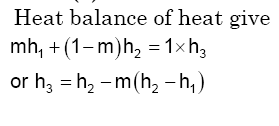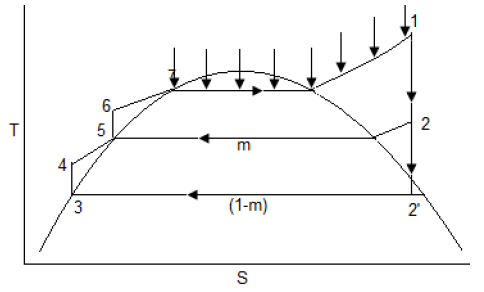QUESTION: 6

In a steam power plant operating on the Rankine cycle, steam enters the turbine at 4 MPa, 350°C and exits at a pressure of 15 kPa. Then it enters the condenser and exits as saturated water. Next, a pump feeds back the water to the boiler. The adiabatic efficiency of the turbine is 90%. The thermodynamic states of water and steam are given in the table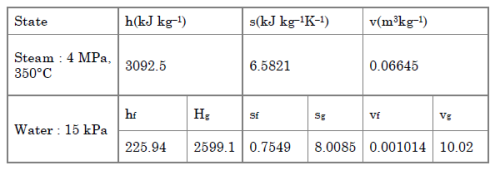h is specific enthalpy, s is specific entropy and v the specific volume; subscripts f and g denote saturated liquid state and saturated vapour state

The net work output (kJ kg-1) of the cycle is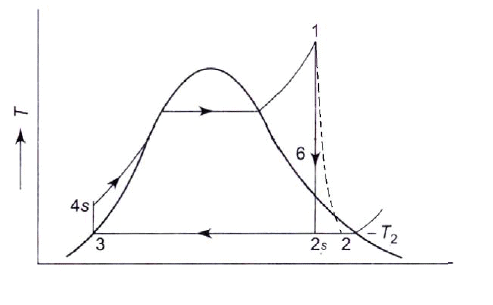Solution: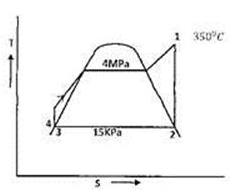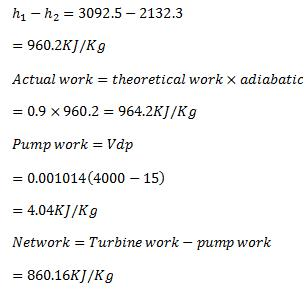QUESTION: 7

In a steam power plant operating on the Rankine cycle, steam enters the turbine at 4 MPa, 350°C and exits at a pressure of 15 kPa. Then it enters the condenser and exits as saturated water. Next, a pump feeds back the water to the boiler. The adiabatic efficiency of the turbine is 90%. The thermodynamic states of water and steam are given in the table.h is specific enthalpy, s is specific entropy and v the specific volume; subscripts f and g denote saturated liquid state and saturated vapour state.

Heat supplied (kJ kg-1) to the cycle is

Solution:

Heat supplied to the cycle = h4  -h2= 3092.5 - 229.98 = 2863 kJ/kg

QUESTION: 8

For a given set of operating pressure limits of a Rankine cycle, the highest, efficiency occurs for

Solution:

Efficiency of ideal regenerative cycle is exactly equal to that of the corresponding Carnot cycle. Hence it is maximum

QUESTION: 9

A thermal power plant operates on a regenerative cycle with a single openfeed water heater, as shown in the figure. For the state points shown, thespecific enthalpies are: h1 = 2800 kJ/kg and h2 = 200 kJ/kg. The bleed to thefeed water heater is 20% of the boiler steam generation rate. The specificenthalpy at state 3 is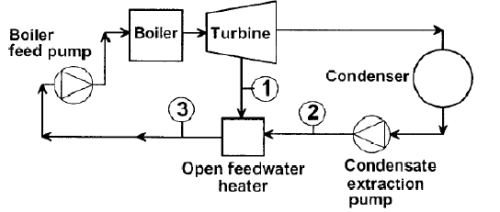Solution: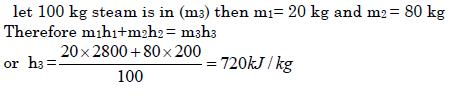QUESTION: 10

For two cycles coupled in series, the topping cycle has an efficiency of 30%and the bottoming cycle has an efficiency of 20%. The overall combinedcycle efficiency is

Solution:

(1 -h) = (1 -h )(1 -h )(1 -h ).................................................... ........... i1 -hm ) or n = n1 + n2 - n1n2 =(0.30 + 0.2 - 0.3 x 0.2)x 100% = 44%

QUESTION: 11

Consider the following processes:

.4. Constant pressure heat rejection.

The correct sequence of these processes in Rankine cycle is::

Solution:
QUESTION: 12

In a Rankine cycle, with the maximum steam temperature being fixed from metallurgical considerations, as the boiler pressure increases

Solution:

with increase in pressure, state of steam shifts towards left and thus on expansion, quality of steam will decrease

QUESTION: 13

In which one of the following working substances, does the relation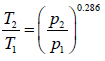hold good if the process takes place with zero heat transfer?

Solution:

zero heat transfer means adiabatic process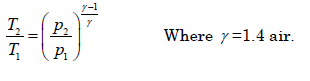QUESTION: 14

Consider the following for a steam turbine power plant:

2. Increase in turbine speed.

3. Increase in specific output.

4. Increase in cycle efficiency.

Which of the above occur/occurs due to reheating of steam?

Solution:

1. Quality of steam improves so blade erosion reduced.

2. Reheating has no effect on speed. So 2 is false.

QUESTION: 15

Blade erosion in steam turbines takes place

Solution:
QUESTION: 16

Consider the following statements The reheat cycle helps to reduce

1. Fuel consumption

2. Steam flow

3. The condenser size

Which of these statements are correct?

Solution:
QUESTION: 17

Consider the following statements regarding effects of heating of steam ina steam turbine:

1. It increases the specific output of the turbine

2. It decreases the cycle efficiency

4. It improves the quality of exit steam?

Which of these statements are correct?

Solution:

Heating of steam increases specific output of turbine and improves the quality of exit steam.

QUESTION: 18

Employing superheated steam in turbines leads to

Solution:
QUESTION: 19

Which one of the following is correct?In ideal regenerative cycle the temperature of steam entering the turbine is same as that of

Solution: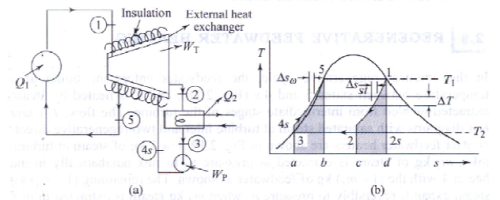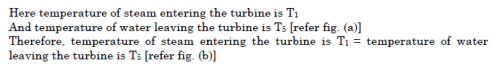QUESTION: 20

In a steam power plant, the ratio of the isentropic heat drop in the primemover to the amount of heat supplied per unit mass of steam is known as

Solution: